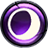◄ Return to the Main Guide Power Calculator Math

You may be wondering how the power calculator computes probabilities. In order to understand the math, we need to discuss binomial coefficients and hypergeometric distributions.

A binomial coeffient (sometimes referred to as "n choose k") is a way of computing the number of ways to select a subset of items from a larger collection. In the context of a card game, we can use it to compute the number of different n card hands drawn from a N card deck.

 ((N),(n)) = (N!)/(n!(N-n)!)

N = Cards in deck
n = Number of cards drawn

While ((N),(n)) will give us the number of different hands possible to draw, it doesn't directly give us any probabilities. However, we can get the probability of drawing exactly k power cards from a deck containing K power cards (of N cards total) by dividing the number of possible hands containing k power cards by the number of total possible hands. This is referred to as a hypergeometric distribution.

 P(k) = (((K),(k))((N-K),(n-k))) / (((N),(n)))

K = Power cards in deck
k = Power cards drawn
N = Total cards in deck
n = Total cards drawn

So, that's useful. P(k) will give us the probability of drawing a hand of exactly k power cards. However, we usually care about the probability of drawing at least k power cards, rather than the probability of drawing exactly k power cards. We can find the probability of drawing at least k power by adding up the probability values for every value of k, up to the maximum number of power cards.

P(k >= T) = sum_(i=T)^(K) P(i)

K =  Power cards in deck
k =  Power cards drawn
T =  Target power cards
n =  Total cards drawn

Okay, so now we know how to compute the probability of drawing enough power. But what if we care about multiple kinds of influence? As it turns out, we can generalize the hypergeometric distribution to compute probabilities when we care about multiple different card types drawn. For example, fire influence drawn and time influence drawn.

 P(k_F,k_T) = (((K_F),(k_F)) ((K_T),(k_T)) ((N-K_F-K_T), (n-k_F-k_T))) / (((N),(n)))

K_F = Fire influence cards in deck
K_T = Time influence cards in deck
k_F = Fire influence cards drawn
k_T = Time influence cards drawn
N = Total cards in deck
n = Total cards drawn

Again, this will give us the probability P(k_F, k_T) of an exact number of fire and time, but we usually care about drawing at least that much, so we'll need to add together all the possible combinations of at least those target numbers.

 P(k_F>=T_F, k_T>=T_T) = sum_(i=T_F)^(K_F) sum_(j=T_T)^(K_T) P(i, j)

Now we're getting somewhere! However, the above math is still a simplified version of what the power calculator actually does. All the above math assumes that individual cards provide one kind of influence, but not more than one kind of influence, or both power and influence. As we know, in reality, cards can provide multiple kinds of things: perhaps power and time influence, or power and time influence and fire influence.

In order to account for this, the Power Calculator first groups cards according to the sorts of resources they produce. After generating these groupings, it will enumerate all possible combinations of those grouping which — when combined — will generate enough power and influence to satisfy the target power and influence cost. For each of those enumerated combinations, it will compute the probability of drawing that specific number of sources from each group. Finally, it will sum those probability to find an overall probability of satisfying the target cost.

As an example, consider the case where we have a deck with three groups of power/influence sources: time sigils (1T), fire sigils (1F), and additional power sources which provide both fire and time (1FT). Now, say we want to find the probability of being able to play a card costing 2FT. We can sum up the possible combination of grouped cards which will provide enough power and influence to satisfy the overall cost:

 P_(2FT) = {: (P(k_(1FT) >= 2, k_(1T) >= 0, k_(1F) >= 0)), (+), (P(k_(1FT) = 1, k_(1T) >= 1, k_(1F) >= 0)), (+), (P(k_(1FT) = 1, k_(1T) = 0, k_(1F) >= 1)), (+), (P(k_(1FT) = 0, k_(1T) >= 1, k_(1F) >= 1)) :}

Thus, the calculator will compute the probability for each specific combination of grouped cards which happens to satisfy the target cost, and then sum those probabilities into an the overall probability value. The Mulligan Phase Deciding whether or not to keep your opening hand is a strategic decision in Eternal. If you choose to re-draw, your starting hand is guaranteed to include either 2, 3 or 4 power cards (with an equal chance of each).

This raises your chances of having more Influence to start with - so (generally speaking) your odds may be ever-so-slightly higher than what is actually depicted on the charts in the Eternal Power Calculator. Pre-requisites On the other hand, some power sources have a Power cost associated with them. Seek Power and the five Favors are the most obvious examples.

The Eternal Power Calculator considers any card that increases your Power to be a Power source when determining probabilities. But it does not consider the sequence in which cards are played.

In other words, including Vara's Favor in your deck will increase the overall chances of reaching your desired Shadow Influence costs. But because it can not be played until Turn 2,  if you draw Vara's Favor instead of a Power card your true odds of achievingon Turn 1 will be ever-so-slightly lower than what is represented on the charts.